Courses

# NCERT Solutions(Part - 2) - Algebra Class 6 Notes | EduRev

## Class 6 : NCERT Solutions(Part - 2) - Algebra Class 6 Notes | EduRev

The document NCERT Solutions(Part - 2) - Algebra Class 6 Notes | EduRev is a part of the Class 6 Course Mathematics (Maths) Class 6.
All you need of Class 6 at this link: Class 6

Exercise 11.4

(a) Take Sarita’s present age to be y years

(i) What will be her age 5 years from now?

(ii) What was her age 3 years back?

(iii) Sarita’s grandfather is 6 times her age. What is the age of her grandfather?

(iv) Grandmother is 2 years younger than grandfather. What is grandmother’s age?

(v) Sarita’s father’s age is 5 years more than 3 times Sarita’s age. What is her father’s age?

(b) The length of a rectangular hall is 4 meters less than 3 times the breadth of the hall. What is the length, if the breadth is b meters?

(c) A rectangular box has height h cm. Its length is 5 times the height and breadth is 10 cm less than the length. Express the length and the breadth of the box in terms of the height.

(d) Meena, Beena and Leena are climbing the steps to the hill top. Meena is at step s, Beena is 8 steps ahead and Leena 7 steps behind. Where are Beena and Meena? The total number of steps to the hill top is 10 less than 4 times what Meena has reached. Express the total number of steps using s.

(e) A bus travels at v km per hour. It is going from Daspur to Beespur. After the bus has travelled 5 hours, Beespur is still 20 km away. What is the distance from Daspur to Beespur? Express it using v.

(a) (i) y + 5

(ii) y - 3

(iii) 6 y

(iv) 6y - 2

(v) 3y + 5

(b) Length = 3b and Breadth = (3b - 4) meters

(c) Height of the box = h cm

Length of the box = 5 times the height = 5h cm

Breadth of the box = 10 cm less than length = (5h - 10) cm

(d) Meena’s position = s

Beena’s position = 8 steps ahead = s + 8

Leena’s position = 7 steps behind = s - 7

Total number of steps = 4s - 10

(e) Speed of the bus = v km/h

Distance travelled in 5 hours = 5v km

Remaining distance = 20 km

Therefore, total distance = (5v + 20) km

Question 2: Change the following statements using expressions into statements in ordinary language.

(For example, Given Salim scores r runs in a cricket match, Nalin scores

(r + 15) runs. In ordinary language − Nalin scores 15 runs more than Salim.)

(a) A note book costs Rs p. A book costs Rs 3 p.

(b) Tony puts q marbles on the table. He has 8 q marbles in his box.

(c) Our class has n students. The school has 20 students.

(d) Jaggu is z years old. His uncle is 4 z years old and his aunt is (4z − 3) years old.

(e) In an arrangement of dots there are r rows. Each row contains 5 dots.

(a) A book cost 3 times the cost of a notebook.

(b) The number of marbles in box is 8 times the marble on the table.

(c) Total number of students in the school is 20 times that in our class.

(d) Jaggu’s uncle’s age is 4 times the age of Jaggu. Jaggu’s aunt is 3 years younger than his uncle.

(e) The total number of dots is 5 times the number of rows.

Question 3: (a) Given Munnu’s age to be x years, can you guess what (x − 2) may show? (Hint: Think of Mannu’s younger brother.)

Can you guess what (x + 4) may show? What (3x + 7) may show?

(b) Given Sara’s age today to be y years. Think of her age in the future or in the past.
What will the following expression indicate?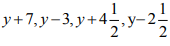(c) Given n students in the class like football, what may 2n show? What may n/2 show? (Hint: Think of games other than football).

Answer 3: (a) Munnu’s age = x years

His younger brother is 2 years younger than him = (x - 2) years

His elder brother’s age is 4 years more than his age = (x + 4) years

His father is 7 year’s more than thrice of his age = (3x + 7) years

(b) Her age in past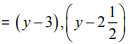Her age in future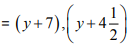(c) Number of students like hockey is twice the students liking football, i.e., 2n

Number of students like tennis is half the students like football, i.e., n/2

Exercise 11.5

Question 1: State which of the following are equations (with a variable). Give reason for your answer. Identify the variable from the equations with a variable.

(a) 17 = x + 7

(b) (t − 7) > 5

(c) 4/2 = 2

(d) (7 × 3) − 19 = 8

(e) 5 × 4 − 8 = 2x

(f) x − 2 = 0

(g) 2m < 30

(h) 2n + 1 = 11

(i) 7 = (11 × 5) − (12 × 4)

(j) 7 = (11 × 2) + p

(k) 20 = 5y

(l)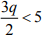(m) + 12 > 24

(n) 20 − (10 − 5) = 3 × 5

(o) 7 − x = 5

(a) It is an equation of variable as both the sides are equal. The variable is x.

(b) It is not an equation as L.H.S. is greater than R.H.S.

(c) It is an equation with no variable. But it is a false equation.

(d) It is an equation with no variable. But it is a false equation.

(e) It is an equation of variable as both the sides are equal. The variable is x.

(f) It is an equation of variable x.

(g) It is not an equation as L.H.S. is less than R.H.S.

(h) It is an equation of variable as both the sides are equal. The variable is n.

(i) It is an equation with no variable as its both sides are equal.

(j) It is an equation of variable p.

(k) It is an equation of variable y.

(l) It is not an equation as L.H.S. is less than R.H.S.

(m) It is not an equation as L.H.S. is greater than R.H.S.

(n) It is an equation with no variable.

(o) It is an equation of variable x

Question 2: Complete the entries in the third column of the table.

 S. No. Equation Value of variable Equation satisfied Yes/No (a) 10y = 80 y = 10 - (b) 10y = 80 y = 8 - (c) 10y = 80 y = 5 - (d) 4l = 20 l= 20 - (e) 4l = 20 l= 80 - (f) 4l = 20 l = 5 - (g) b + 5 = 9 b = 5 - (h) b + 5 = 9 b = 9 - (i) b + 5 = 9 b = 4 -
 (j) h - 8 = 5 h = 13 - (k) h - 8 = 5 h = 8 - (l) h - 8 = 5 h = 0 - (m) p + 3 = 1 p= 3 - (n) p+ 3 =1 p = 1 - (o) p+ 3 = 1 P = 0 - (p) p+ 3 = 1 P = - 1 - (q) p + 3 = 1 P = -2 -

 S. No. Equation Value of variable Equ. satisfied Yes / No Sol. of L.H.S. (a) 10y = 80 y = 10 No 10 x 10 = 100 (b) 10y = 80 y = 8 Yes 10 x 8 = 80 (c) 10y = 80 y = 5 No 10 x 5 = 50 (d) 4l= 20 l = 20 No 4 x 20 = 80 (e) 4l = 20 l = 80 No 4 x 80 = 320 (f) 4l = 20 l = 5 Yes 4 x 5 = 20 (g) b + 5 = 9 b = 5 No 5 + 5 = 10 (h) b + 5 = 9 b = 9 Yes 9 + 5 = 14 (i) b + 5 = 9 b = 4 Yes 4 + 5 = 9 (j) h - 8 = 5 h = 13 Yes 13 - 8 = 5 (k) h - 8 = 5 h = 8 No 8 - 8 = 0 (l) h - 8 = 5 h = 0 No 0 - 8 = -8 (m) No 3 + 3 = 6 (n) p + 3 = 1 P = 3 No 1 + 3 = 4 (o) p+3 = 1 P = 1 No 0 + 3 = 3 (P) p+3 = 1 P = 0 No -1 + 3 = 2 (q) p+3 = 1 p = -1 Yes -2 + 3 = 1 p+3 = 1 p = - 2

Question 3: Pick out the solution from the values given in the bracket next to each equation. Show that the other values do not satisfy the equation.

(a) 5m = 60 (10, 5, 12, 15)

(b) n + 12 = 20 (12, 8, 20, 0)

(c) p − 5 = 5 (0, 10, 5 − 5)

(d) q/2 =  (7, 2, 10, 14)

(e) r − 4 = 0 (4, − 4, 8, 0)

(f) + 4 = 2 (− 2, 0, 2, 4)

Answer 3: (a) 5m = 60

Putting the given values in L.H.S.,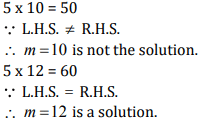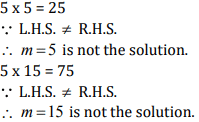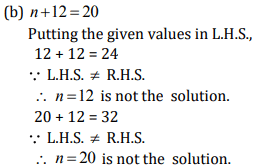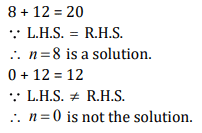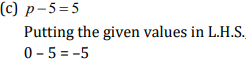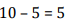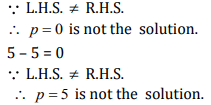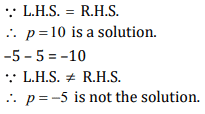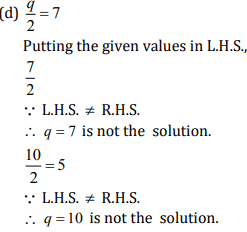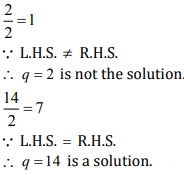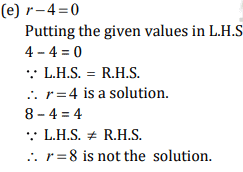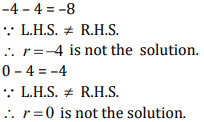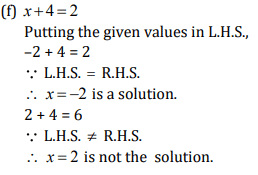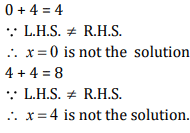Question 4: (a) Complete the table and by inspection of the table, find the solution to the equation m + 10 = 16.

 m 1 2 3 4 5 6 7 8 9 10 — — — m + 10 — — — — — — — — — — — — —

(b) Complete the table and by inspection of the table, find the solution to the equation 5t = 35.

 t 3 4 5 6 7 8 9 10 11 — — — — — 5t — — — — — — — — — — — — — —

(c) Complete the table and find the solution of the equation z/3 = 4 using the table.

 z 8 9 10 11 12 13 14 15 16 — — — — z/3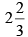3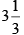— — — — — — — — — —

(d) Complete the table and find the solution to the equation m − 7 = 3

 m 5 6 7 8 9 10 11 12 13 — — m - 7 — — — — — — — — — — —

(a)

 m 1 2 3 4 5 6 7 8 9 10 11 12 13 m + 10 11 12 13 14 15 16 17 18 19 20 21 22 23

∵ At m = 6, m +10 = 16
∴ m = 6 is the solution.

(b)

 t 3 4 5 6 7 8 9 10 11 12 13 14 15 16 5t 15 20 25 30 35 40 45 50 55 60 65 70 75 80

∵ At t = 7, 5t = 35
∴  t = 7 is the solution.
(c)

 z 8 9 10 11 12 13 14 15 16 17 18 19 20 z/3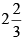3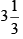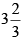4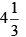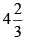5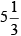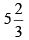6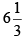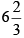∵ At z = 12, z/3 = 4
∴ z = 12 is the solution.

(d)

 m 5 6 7 8 9 10 11 12 13 14 15 m - 7 -2 -1 0 1 2 3 4 5 6 7 8
Offer running on EduRev: Apply code STAYHOME200 to get INR 200 off on our premium plan EduRev Infinity!

## Mathematics (Maths) Class 6

185 videos|229 docs|43 tests

,

,

,

,

,

,

,

,

,

,

,

,

,

,

,

,

,

,

,

,

,

;